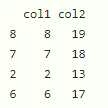# How to Generate a Sample Using the Sample Function in R?

• Last Updated : 19 Dec, 2021

In this article, we will discuss how to generate a sample using the sample function in R.

Sample() function is used to generate the random elements from the given data with or without replacement.

Syntax:

`sample(data, size, replace = FALSE, prob = NULL)`

where,

• data can be a vector or a dataframe
• size represents the size of the sample
• replace is used to set the values again repeated if it is set to true
• prob: a vector of probability weights for obtaining the elements of the vector being sampled

### Example 1: Generate sample data from the vector

Here, we will generate the n sample data from the given vector with 11 elements using the sample function.

## R

 `# consider the vector``data=``c``(23,45,21,34,5,6,7,8,86,45,3)`` ` `# get 4 random elements``print``(``sample``(data,4))`` ` `# get 1 random element``print``(``sample``(data,1))`` ` `# get 6 random elements``print``(``sample``(data,6))`

Output:

``` 45  7  5 34
 3
  5 23  8 21  6 45```

### Example 2: Generate sample data from the vector by replacing

Here we are going to create a vector with 11 elements and generate the sample data with a replacement.

## R

 `# consider the vector``data=``c``(23,45,21,34,5,6,7,8,86,45,3)`` ` `# get 4 random elements``print``(``sample``(data,4,replace=``TRUE``))`` ` `# get 1 random element``print``(``sample``(data,1,replace=``TRUE``))`` ` `# get 6 random elements``print``(``sample``(data,6,replace=``TRUE``))`

Output:

``` 45  5  5  3
 86
  5  5  8  7  8 45```

### Example 3: Sampling with Uneven Probabilities Using sample Function

Here we are going to select the elements with higher probability than others by setting the probability using the prob parameter.

## R

 `# consider the vector``data=``c``(23,45,21,34,5)`` ` `# get 10 random elements with probability``print``(``sample``(data, size = 10, replace = ``TRUE``, ``             ``prob = ``c``(0.6,0.1,0.1,0.1,0.1)))`

>

Output:

`  23 23 23 23 23 45 23 23 23 23`

### Example 4: Random Sampling of Data Frame Rows Using sample Function

Here we are going to sample the dataframe, let us create a dataframe and sample the rows.

## R

 `# create dataframe with 2 columns``data=``data.frame``(col1=``c``(1:10),col2=``c``(12:21))`` ` `# get the sample of 4 in each column``data[``sample``(1:``nrow``(data), size = 4), ]`

Output:### Example 5: Random Sampling of List Elements Using sample Function

Here we are going to sample the data in the list  with size 4

## R

 `# create list with some integers``data=``list``(1,2,3,4,5,6)`` ` `# get the sample of 4``data[``sample``(1:``length``(data), size = 4)]`

Output:

```[]
 2

[]
 1

[]
 4

[]
 6```

My Personal Notes arrow_drop_up